# While Loop C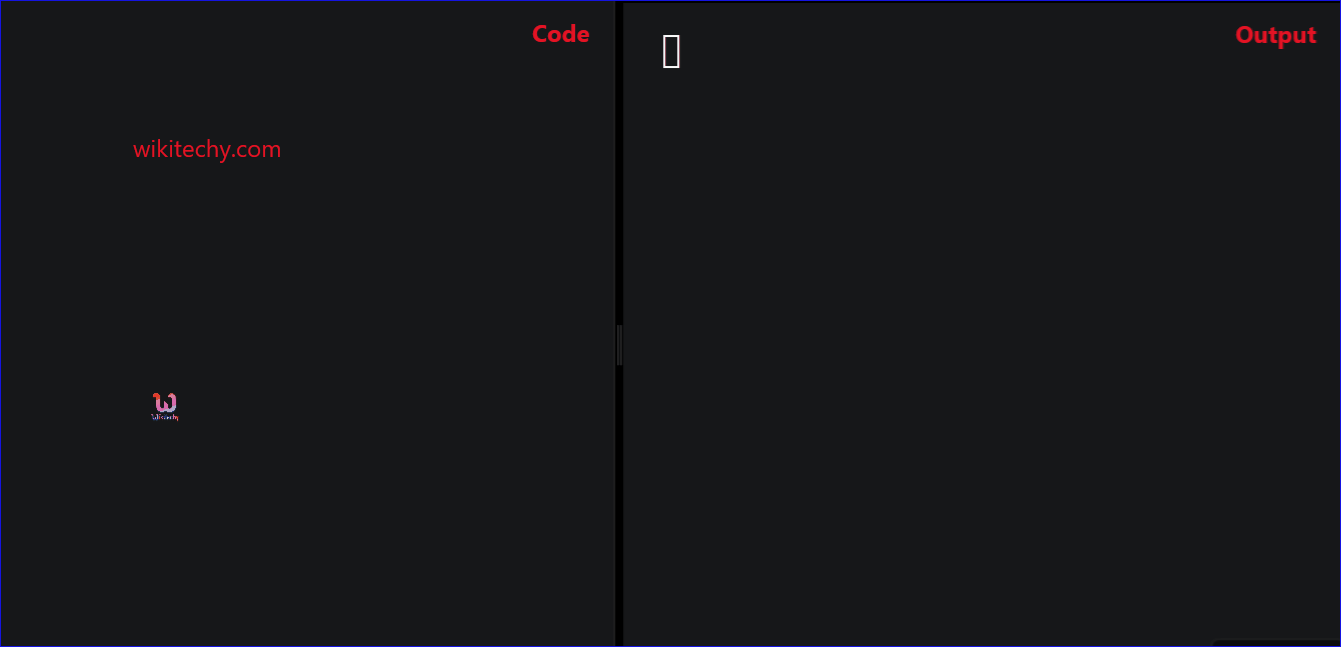Learn C - C tutorial - While loop c - C examples - C programs

## While Loop C - Definition and Usage

• In C- Programming the while statement is considered as a looping statement.
• In C , the while Loop is executed, only when condition is true.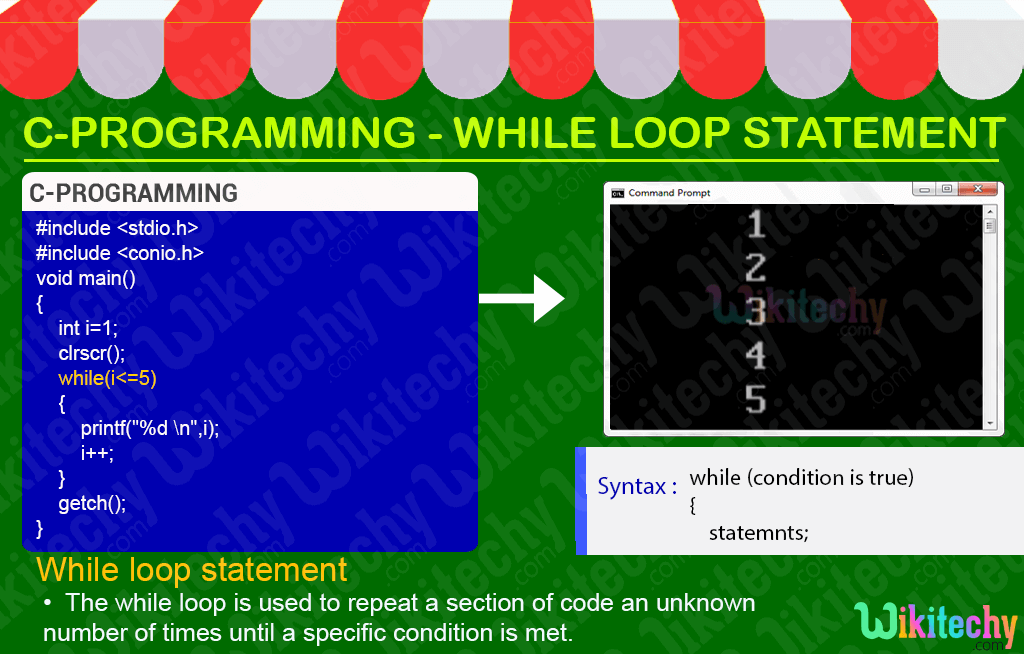## C Syntax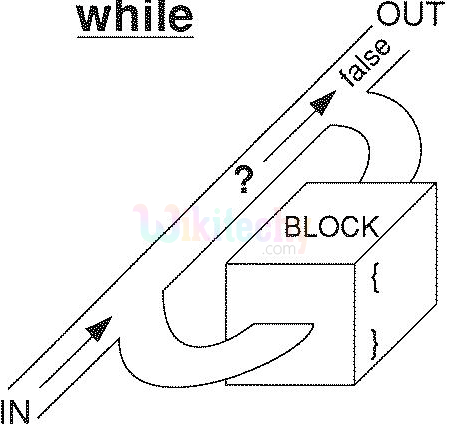## C Code - Explanation :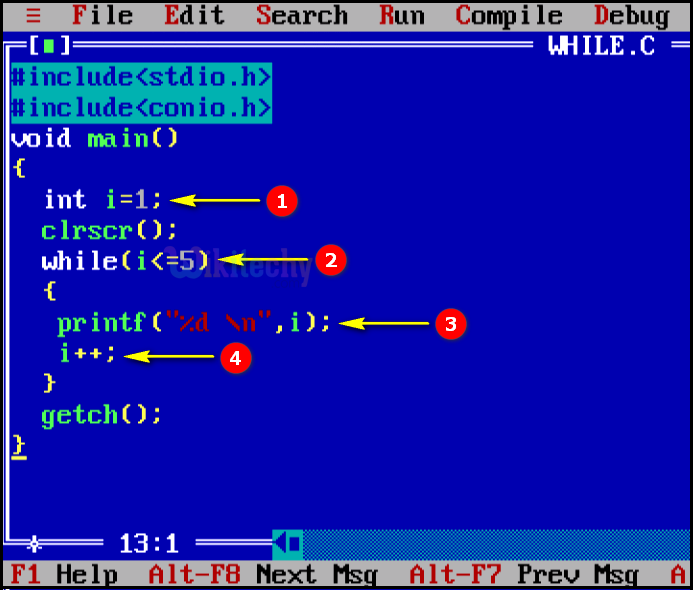1. Here in this statement we declare and initialize the value of “i=1”.
2. In this statement we are defining the while statement and the condition for the execution of “i” value which is less than are equal to “5”, where it executes the statement.
3. In this statement we print the value of “i”.
4. Here in this statement the “i” value is incremented by one for each loop execution.

## Sample Output - Programming Examples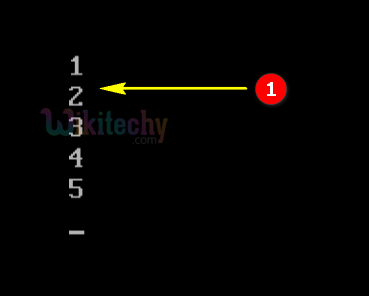1. Here in this output the “i” value is incremented by one for each while loop execution which will be executed until the “i” value is less than are equal to “5”.